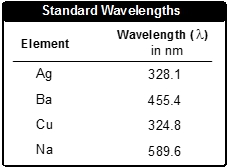## General Chemistry

Learn the toughest concepts covered in Chemistry with step-by-step video tutorials and practice problems by world-class tutors

9. Quantum Mechanics

# Speed of Light

The Speed of Light in a vacuum is related to the wavelength and frequency of a light particle.

Speed of Light
1
concept

## Speed of Light57s
Play a video:
So here we say the speed of light is the product of wavelength and frequency, and in a vacuum it travels at the speed of 3.0 times 10 to the 8 m per second. We're going to say here it is a physical constant and uses the variable See, So we know that C now represents the speed of like and with it we have our speed of light formula here, see, our speed of light equals wavelength, which we said was lambda Times are frequency which we said was meal. So here, we're going to say Remember, frequency uses mu and wavelength uses lambda and remember, frequency is in units of seconds in verse which is equivalent or equal to hurts. And that wavelength is in units off meters. Remember, it's in this in these units, so that matches the units off our speed of light. Now that we know how wavelength and frequency are connected to the speed of light, move onto the next video and take a look at the example question
2
example

## Speed of Light Example 11m
Play a video:
in this example, it's as to calculate the frequency of the red light emitted by a neon sign with a wavelength of 663.8 nanometers. All right, so we need to determine what our frequency is, which is Mu. They're giving us our wavelength, which is Lambda. Remember, the equation that connects them together is that the speed of light equals them multiplying one another. Now, remember, wavelength needs to be in units off meters. So we need to do a metric prefix conversion where we have 663. nanometers on. We're going to say here that for every one nanometer, it's 10 to the negative 9 m. So here meters will come out to be 6.638 times 10 to the negative 7 m. Now that we have that, we can plug it in. We have the speed of light. We have. We're looking for frequency. We have our wave length in meters, so divide both sides now by meters. Yeah, So when we do that, we're gonna get left at the end. Are seconds inverse. So here we're gonna say frequency equals four point 519 times 10 to the seconds inverse. Because, remember, meters, cancel out here. So we're left with one over seconds, which is the same thing as seconds inverse. Okay, so you're units. Could be either one over seconds or seconds. Inverse or hurts. All of them mean the same thing. And your answer has four sig figs. Because our wavelength was given to us also within four sig figs.
3
Problem

Even the music we listen to deals with how energy travels to get to our car radio. If an FM Radio station broadcasts its music at 97.7 MHz find the wavelength in angstroms of the radio waves. One angstrom is equal to 10-10 m.

4
Problem

The distance between the earth and the sun is 1.496 x 1017 μm. How long (in mins) will it take for light to go from the sun to earth?

5
Problem

When they are burned, certain elements emit light at a specific wavelength. Some wavelengths for certain elements are provided below:When burned, an unknown element emits light at a frequency of 9.23 x 1014 s-1. What is the identity of this unknown element?